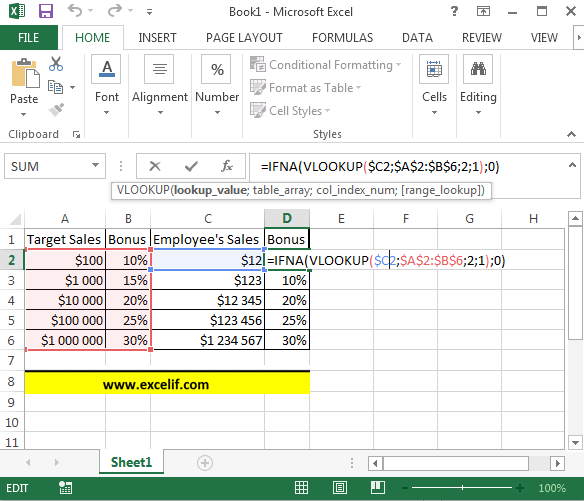### Vlookup For A Range

Doing vlookup for a range of values is also possible. Here you have an example which shows you how to deal with vlookup for a range in Excel.

Your company set the new targets of sales. If employee reached the target, he will get a bonus. The higher target is the higher bonus. Let’s calculate the bonus using vlookup function.

=IFNA(VLOOKUP(\$C2,\$A\$2:\$B\$6,2,1),0)Explanation:

C2 – here is the lookup value

A2:B6 – this is a whole range of data (table_array)

2 – number of column in the range

1 – stands for approximate match

IFNA – this function checks if there is some bonus. If not it writes \$0.

## Template

```Further reading: